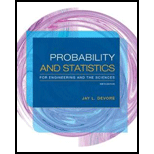# A branch of a certain bank in New York City has six ATMs. Let X represent the number of machines in use at a particular time of day. The cdf of X is as follows: F ( x ) = { 0 x &lt; 1 .60 0 ≤ x &lt; 1 .19 1 ≤ x &lt; 2 .39 2 ≤ x &lt; 3 .67 3 ≤ x &lt; 4 .92 4 ≤ x &lt; 5 .97 5 ≤ x &lt; 6 1 6 ≤ x Calculate the following probabilities directly from the cdf: a. p (2), that is, P ( X = 2) b. P ( X &gt; 3) c. P (2 ≤ X ≤ 5) d. P (2 &lt; X &lt; 5)### Probability and Statistics for Eng...

9th Edition
Jay L. Devore
Publisher: Cengage Learning
ISBN: 9781305251809### Probability and Statistics for Eng...

9th Edition
Jay L. Devore
Publisher: Cengage Learning
ISBN: 9781305251809

#### Solutions

Chapter
Section
Chapter 3.2, Problem 23E
Textbook Problem

## A branch of a certain bank in New York City has six ATMs. Let X represent the number of machines in use at a particular time of day. The cdf of X is as follows: F ( x )  =  { 0   x < 1 .60  0 ≤ x < 1 .19  1 ≤ x < 2 .39 2 ≤ x < 3 .67  3 ≤ x < 4 .92 4 ≤ x < 5 .97  5 ≤ x < 6 1 6 ≤ x Calculate the following probabilities directly from the cdf: a. p(2), that is, P(X = 2) b. P(X > 3) c. P(2 ≤ X ≤ 5) d. P(2 < X < 5)

Expert Solution

a.

To determine

Find P(X=2).

### Explanation of Solution

Given info:

In New York, a branch of a certain bank has 6 ATMs. Let X be the number of machines in use at a particular time of the day. The cdf is given by:

F(x)={0, if x<00.06, if 0x<10.19, if 1x<20.39, if 2x<30.67, if 3x<40.92, if 4x<50

Expert Solution

b.

To determine

Find the value of P(X>3)

Expert Solution

c.

To determine

Find the value of P(2X5)

Expert Solution

d.

To determine

Find the value of P(2<X<5)

### Want to see the full answer?

Check out a sample textbook solution.See solution

### Want to see this answer and more?

Bartleby provides explanations to thousands of textbook problems written by our experts, many with advanced degrees!

See solution